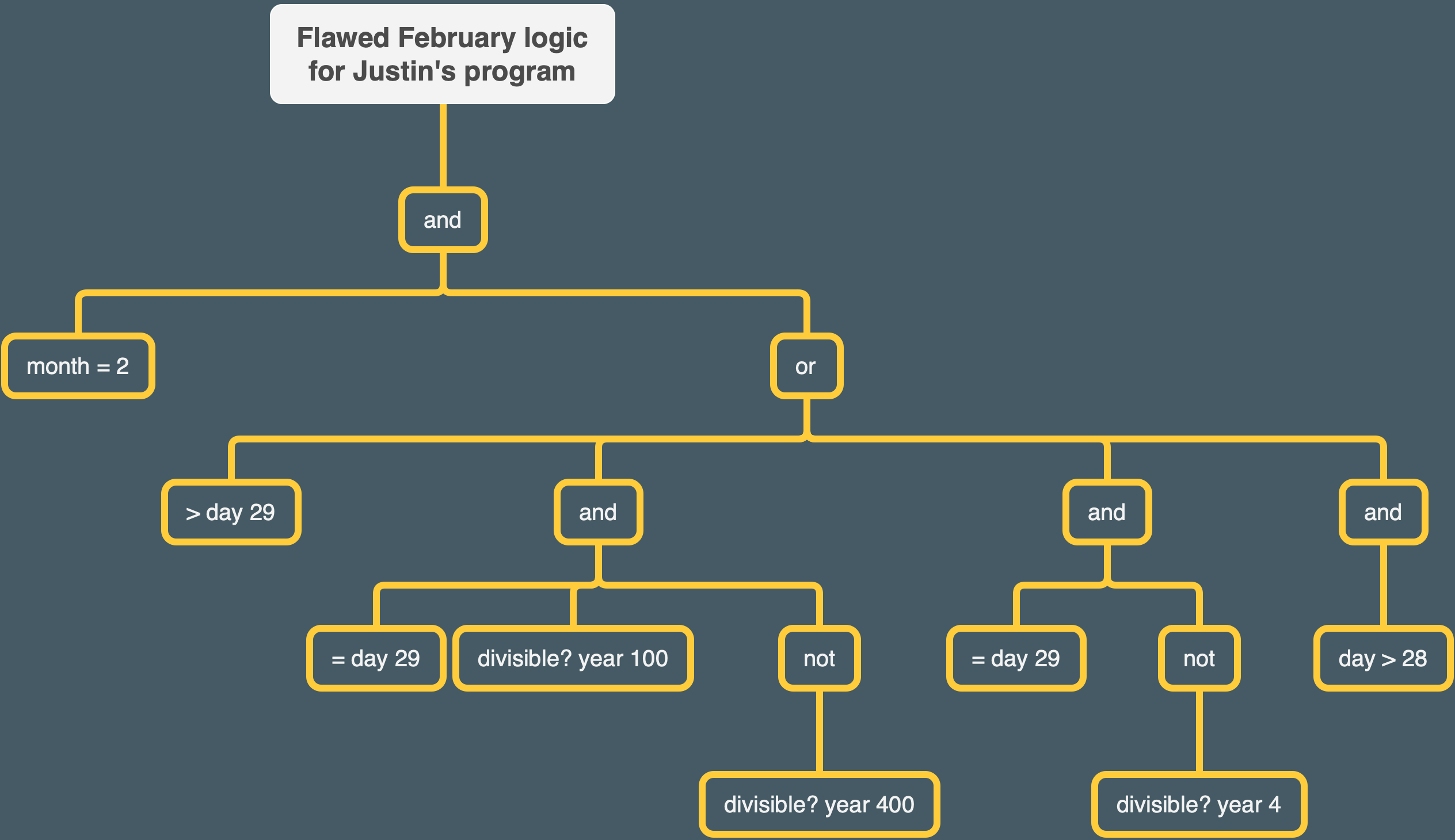# Simply Scheme Chapter 6 Error Follow-up

I caught an error in my solution for Exercise 6.11 for Simply Scheme Chapter 6. I noticed that there didn’t seem to be the correct number of matching parentheses on one line. Once I corrected the error, the function started failing one of my tests. So the passing was just through happenstance. I’m going to put the parentheses error aside and focus on what I consider to be the basic logical mistake.

Here is the erroneous procedure with the parentheses that I intended:

For this procedure, `(valid-date? 2 29 1964)` returns false but i want it to return true. What’s the problem?The problem is the manner in which I constructed the logic. If anything nested under the “or” returns true, then the whole February-related line of the `cond` will return false. So let’s run through the program with the example the input I’m having trouble with, `2 29 1963`.

Since the day is = 29, `(> day 29)` returns false. So far so good.
Since the year is not divisible by 100, `(and (= day 29)(divisible? year 100) (not (divisible? year 400))) ` returns false.
Since the year is divisible by 4, `(and (= day 29)(not (divisible? year 4)))` returns false.
The day is > 29, though, so `(and (> day 28)))))` returns true. Uh-oh.

So what’s going wrong?

I think for this part of my program to make sense, all the things under the “or” have to be stuff that I never want to happen.

For Februaries, the number of days is never greater than 29. So `(> day 29)` is okay.

For Februaries, you can never have a situation where the number of days is 29, and the year is divisible by 100 but not 400, so `(and (= day 29)(divisible? year 100) (not (divisible? year 400))) ` is okay.

You can never have a situation where February has 29 days and the year isn’t divisible by 4 (some cases where the year is divisible by 4 and the date is still invalid exist, but those are covered by the rule in the last paragraph) . So `(and (= day 29)(not (divisible? year 4)))` is okay.

Re: `(and (> day 28))`, there are years that I want to return as valid even when they have more than 28 days in Febraury — specifically, the leap years. But this part of the program will be checked every time `(valid-date?)` is run, and so every leap year date will fail.

The `(and (> day 28))` is actually introducing an entirely unnecessary error. The program already covers the prohibited cases for February. Days being equal to, for example, 30 is entirely prohibited under the rule that says days can never be more than 29, and days being equal to 29 is prohibited in the cases not allowed by the rules on leap years. So what’s left for `(and (> day 28))` to do? Nothing except mess up my program!

Here is my fixed procedure:

## Things to Do Better to Avoid This Kind of Mistake

1. Break up tricky logical things into separate lines of a `cond` rather than trying to be elegant and getting myself confused.
2. Carefully check each line of a program for parentheses issues when I’m done writing.
3. Do more step by step logical analysis of programs using some example inputs and trees.
4. If you’re gonna do “return false if X, else return true” style of organizing a program, consider expressly listing out every prohibited case and thinking about the logical relationships / Venn diagrams etc between the cases.